## LetsPlayMaths.Com

WELCOME TO THE WORLD OF MATHEMATICS

# Class-8 Set Operation

Introduction to Set Operation

Union of Sets

Intersection of Sets

Difference of Two Sets

Complement of Set

Overlapping Set

Disjoint Set

Properties of Set Operation

Identity

Idempotent

Complement

Commutative Property

Associative Property

Distributive Property

Some Facts About Cardinal Number of Set

Set Operation Worksheets

## Introduction to Set Operation

We have already learnt about the basic concept of sets, representing a set by roster or tabular form or by using set builder form, equal set, finite set, infinite sets, and empty set, cardinal number of a finite set, universal set , equivalent sets and sub sets in previous classes. Now we will study about operation on set.

• Union and intersection of sets
• Difference of two sets
• Complement of a set
• Overlapping and disjoint sets
• Basic result about set's cardinal number

## Union of Sets

The union of two sets P and Q is the set consisting of all those elements which belong to either P or Q or both. It is written as (P ∪ Q).

Thus, P ∪ Q = {x|x ∈ P or x ∈ Q}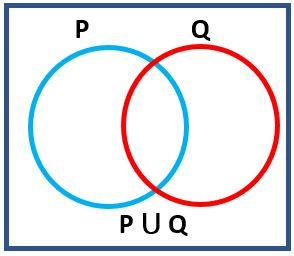Example. P = {1, 2, 3, 4, 5}

Q = {2, 3, 8, 9}

Then, (P ∪ Q) = {1, 2, 3, 4, 5, 8, 9}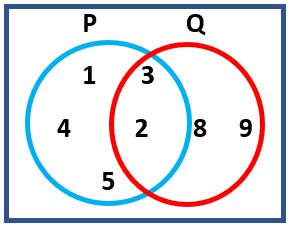## Intersection of Sets

The set consisting of all those elements which belong to both set P and Q and it is called intersection of two sets P and Q. It is written as P ∩ Q. It is read as P intersection Q.

Hence, P ∩ Q = { x| x ∈ P and x ∈ Q}

Example. If P = {a, e, f, g} and Q = {e, f, h, i)

Then, P ∩ Q = {e, f}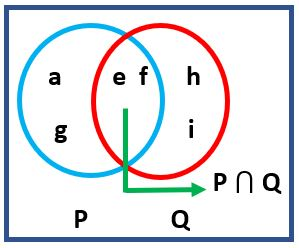## Difference of Two Sets

Difference of two set consist of all those elements which belong to one set but do not belong to other. It is written as P − Q.
Hence, P − Q = {x|x ∈ P and x ∉ Q}
Similarly, for Q − P = {x|x ∈ Q and x ∉ P}
So, P − Q is the set consisting of element of P only and Q − P is the set of consisting of elements of Q only.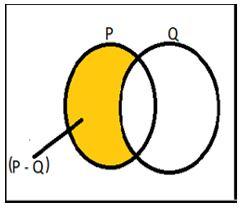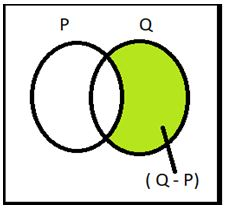Example. If P = {0, 4, 5, 6, 7} and Q = {4, 5, 9, 10, 11}

P − Q = {0, 6, 7}, here 0, 6, 7 are elements present in set P only and not present in Q.

Q − p = {9, 10, 11}, here 9, 10, 11 are elements present only in set Q and not present in set P

## Complement of Set

If P is any set and U is the universal set then the complement of P is the set consisting of all the elements of universal set and do not belong to set P. It is written as P'.

Thus P' = {x|x ∈ ∪ and x ∉ P}

Example. If P = {1, 2, 3, 6, 9} and U = {1, 2, 3, 4, 5, 6, 7, 8, 9, 10}

P' = {4, 5, 7, 8, 10}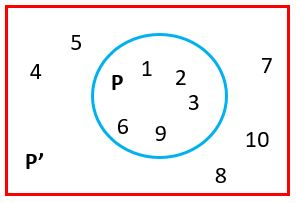## Overlapping Set

Two set said to be overlapping set, if they have at least one element is common. In other way, two sets are overlapping set if P ∩ Q ≠ ∮

Example. Set P = {2, 4, 5, 8, 9} and Set Q = {1, 4, 5, 8, 10, 11}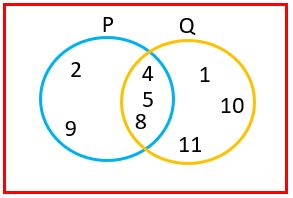Here, set P and set Q are overlapping sets because they have elements 4, 5, and 8 are common. So, here P ∩ Q = {4, 5, 8} ≠ ∮

## Disjoint Set

If two sets have no common element, then it is said to be disjoint set. Two set P and Q are disjoint if P ∩ Q = ∮

Example. The set P = {February, April, may, June} and Set Q = {Sunday, Monday, Tuesday, Wednesday}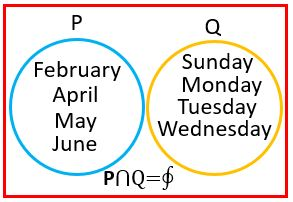Here, these two sets are disjoint set because they have no common element.

## Properties of Set Operation

1. Identity Property
2. Idempotent Property
3. Complement Property
4. Commutative Property
5. Associative property
6. Distributive property

## Identity Property

If P is any set, then

P ∪ ∅ = P

P ∩ U = P

## Idempotent Property

If P is any set, then

P ∩ P = P

P ∪ P = P

## Complement Property

P ∪ P' = U

P ∩ P' = ∅

## Commutative Property

Intersection and union of sets follow the commutative property.

A ∩ B = B ∩ A

A ∪ B = B ∪ A

## Associative Property

Intersection and union of sets follow the associative property.

(A ∩ B) ∩ C = A ∩ (B ∩ C)

(A ∪ B) ∪ C = A ∪ (B ∪ C)

## Distributive Property

Intersection and union of sets follow the distributive property.

A ∪ (B ∩ C) = (A ∪ B) ∩ (A ∪ C)

A ∩ (B ∪ C) = (A ∩ B) ∪ (A ∩ C)

Let's see some solved examples to understand the properties better.

Example 1.If P = {4, 6, 8, 11, 13, 16, 20 ,22, 25}
Q= { 7, 9 , 11,16, 18 , 22,25}
R = { 0, 5, 9, 18,20, 25}
Find i) P ∪ Q
ii) P ∪ R
iii) P ∩ Q
iv) Q ∩ R
v) Q ∪ R
VI) P ∩ R

Solution. i) P ∪ Q ={4, 6, 8, 11, 13, 16, 20, 22, 25, 7, 9, 18, 20}

ii) P ∪ R = {4, 6, 8, 11, 13, 16, 20, 22, 25, 0, 5, 9, 18)

iii) P ∩ Q = {11, 16, 22, 25}

iv) Q ∩ R = {9, 18, 25}

v) Q ∪ R = {7, 9, 11, 16, 18, 22, 25, 0, 5, 20}

vi) P ∩ R = {20, 25}

Example 2. If universal set U = {10, 20, 30, 40, 50, 60, 70, 80, 90} and set A = { 40, 60, 80, 90}, then find A'.

Solution. Complement of A = A' ={10, 20, 30, 50, 70}

Example 3. If Set A = {BHUBANESWAR} and Set B = {AHMEDABAD},
Find i) A ∪ B     ii) A ∩ B     iii) A − B     IV) B − A

Solution Roster form of set A = {B, H, U, A, N, E, S, W, R}
Roster form of set B = {A, H, M, E, D, B}

i) A ∪ B = {B, H, U, A, N, E, S, W, R, M, D}

ii) A ∩ B = {B, H, A, E}

iii) A − B = {U, N, S, W, R}

iv) B − A = {M, D}

## Some Facts About Cardinal Number of Set

If P and Q are two finite sets, then

• n(P ∪ Q) = n(P) + n(Q) − n(P ∩ Q)
• n(P − Q ) = n(P ∪ Q) − n(Q) = n(P) − n(P ∩ Q)
• n(Q − P ) = n(P ∪ Q) − n(P)= n(Q) − n(P ∩ Q)
• n(P ∪ Q) = n(P − Q) + n(Q − P) + n( P ∩ Q)
If universe set is finite set and P is any set, then n(P) + n(P') = n(U) Let's see some solved examples by using cardinal numbers facts

Example 1. If n(P) = 18, n(Q) = 14, and n(P ∪ Q) = 20 ,Then find n(P ∩ Q)

Solution. n(P ∪ Q) = n(P) + n(P ) − n(P ∩ Q)

=> 20 = 18 + 14 − n(P ∩ Q)

=> n(P ∩ Q) = 32 − 20 = 12

Example 2. n(U) = 30, n(P') =12, n(Q) =15, n(P ∩ Q) = 10, Find
i) n(P')
ii) n(P U Q)

Solution.
i) n(Q') = n(U) − n(Q)
= 30 − 15 = 15

ii) n(P ∪ Q) = n(P) + n(Q) − n( P ∩ Q)

here, we first find out n(P) by using formulae

n(P') = n(U) − n(P)

=> 12 = 30 − n(P)

=> n(P) = 18

Then, we put the value of n(P) below equation

n(P ∪ Q) = 18 + 15 − 10=23

Example 3. If n(U)= 50, n(P)= 30, n(Q)= 10 and n{(P ∪ Q)'} = 18, then find

i) n(P ∪ Q )
ii) n( P ∩ Q)
iii) n(P − Q)

Solution.
i) We know n(P ∪ Q) + n{(P ∪ Q)'} = n(U)

=> n(P ∪ Q) + 18 = 50

=> n(P ∪ Q) = 50 − 18 = 32

ii) n(P ∪ Q) = n(P) + n(Q) − n(P ∩ Q)

=> 32 = 30 + 10 − n(P ∩ Q)

=> n(P ∩ Q) = 40 − 32 = 8

iii) n(P − Q ) = n(P) − n(P ∩ Q)

=> n(P − Q ) = 30 &minus 8 = 22

## Class-8 Set Operation Worksheets

Set Operation Worksheet - 1

Set Operation Worksheet - 2